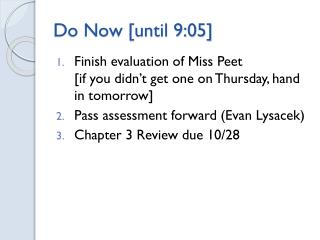DownloadDownload PresentationDo Now [until 9:05]

# Do Now [until 9:05]

Download Presentation## Do Now [until 9:05]

- - - - - - - - - - - - - - - - - - - - - - - - - - - E N D - - - - - - - - - - - - - - - - - - - - - - - - - - -
##### Presentation Transcript

1. Do Now [until 9:05] • Finishevaluation of Miss Peet[if you didn’t get one on Thursday, hand in tomorrow] • Pass assessment forward (Evan Lysacek) • Chapter 3 Review due 10/28

2. Aim: What is weight?

3. Recall • g represents… an acceleration (due to gravity) • Newton’s Second Law: F = ma • What if a = g?

4. F = ma = mg = Fgravity • Fg(gravitational force) • Fg = weight • If gsome other planet instead of gearth, would mass change? Would Fg change? • mass ≠ weight [kg] [N (not SI: pounds)]

5. On Earth, a scale shows that you weigh 495 N. (a) What is your mass? (b) What would the scale read on the moon? (gmoon = 1.60 m/s2) Fg= mg (a) On Earth (b) On Moon m = Fg/ g m = _495 N_ 9.8 m/s2 m = 50.5 kg

6. Spring Scales Fscale= -Fg Scale only reads upward force Fg = mg

7. Fnet Fscale a Fg

8. Fscale a Fnet Fg

9. SNAP! Apparent weight = 0 “weightlessness” a=g Fnet Fg

10. Acceleration while on a scale gives inaccurate reading of weight • Apparent weight: force exerted by scale Fnet = Fscale + Fg

11. Your mass is 83 kg, and you are standing on a scale in an elevator. Starting from rest, the elevator accelerates upward at 3.5 m/s2. (a) What is the scale reading when the elevator is at rest? (b)What is the scale reading when the elevator is accelerating? Fnet = Fscale + Fg • Given: • m = 83 kg • g = 9.8 m/s2 • In (a), elevator at rest, so a = 0 m/s2 • In (b), elevator accelerating upward, so a = 3.5 m/s2 Fnet = Fscale + (-Fg) ma = Fscale – mg Fscale= ma + mg

12. Your mass is 83 kg, and you are standing on a scale in an elevator. Starting from rest, the elevator accelerates upward at 3.5 m/s2. (a) What is the scale reading when the elevator is at rest? (b)What is the scale reading when the elevator is accelerating? For upward accelerating elevator: Fscale= ma + mg (a) (b) Fscale= m(0) + mg Fscale= ma + mg Fscale= m(a + g) Fscale = mg Fscale= (83 kg)[(3.5m/s2) + (9.8m/s2)] Fscale= (83 kg)(9.8 m/s2) Fscale= 1103.9 N Fscale= 813.4 N

13. Alex is on the wrestling team and has a mass of 85.3 kg. Being a whiz at physics, he realizes that he is over the 830 N cutoff for his weight class. If he can convince the trainers to measure his weight in the elevator, what must be the acceleration of the elevator so that he just makes his weight class?

14. An 894 kg dragster, starting from rest, attains a speed of 29.2 m/s in 0.47 s. (a) Find the average acceleration of the dragster during this time interval. (b) What is the magnitude of the average net force on the dragster during this time? (c) Assume that the driver has a mass of 66 kg. What horizontal force does the seat exert on the driver?

15. A grocery sack can withstand a maximum of 230 N before it rips. Will a bag holding 15 kg of groceries that is lifted from the checkout counter at an acceleration of 7.0 m/s2 hold?

16. 71. A 0.60 kg fishbowl is lifted from the ground. What is the smallest force needed to lift it? Describe its resulting motion.

17. Drag Force • Force exerted by fluid (air or water) on object moving through fluid • Ex: air resistance • Opposes motion • Increases with object’s velocity

18. Object in free fall • No vi • Accelerating downward, a = g t = 0 s v = 0 m/s t = 1 s v = 9.8 m/s t = 2 s v = 19.6 m/s t = 3 s v = 29.4 m/s

19. Reach a point where Fnet = 0 • Fdrag = -Fg • Object stops accelerating • Continues to move with constant velocity • Terminal velocity

20. Hannah is going skydiving for her birthday. She has a mass of 68 kg, and jumps from rest. She reaches terminal velocity after falling for 15 seconds. (a) What drag force is exerted on her when she reaches terminal velocity? (b) What is her terminal velocity?